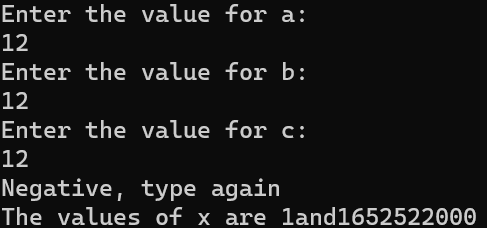# Homework Help for c++ school assessment

``````#include <iostream>
using namespace std;

int equation(int sub, int b)
{
int add = -b + sub;
int div = add / 2;

int subt = -b - sub;
int ide = subt / 2;

return ide;
return div;
}

int main(int div, int ide)
{
int a, b, c;
cout << "Enter the value for a: " << endl;
cin >> a;
cout << "Enter the value for b: " << endl;
cin >> b;
cout << "Enter the value for c: " << endl;
cin >> c;

int doubb = b * b;
int ac = 4 * a * c;
int sub = doubb - ac;
if(sub>=0)
{
equation(sub, b);
}
else
{
cout << "Negative, type again" << endl;
}

cout << "The values of x are " << div << "and" << ide;
}

``````

The main idea of this code is to find the quadratic equation, but i have there seems to be something wrong because the answer is supposed to be just the answer, but instead i got this:I’ve been fixing this code for hours and still get nothing. What is the problem?

You can’t return from a function twice

This cout happens so matter what the value of ‘sub’ is

1 Like